What really the “increasing mass” in relativity means?

Nimit Theeraleekul B. Eng. (Communication)

(1 May 2012)

According to Einstein special theory of relativity (STR), mass seems to increase when it is moving. Anyway, the increasing mass is the prediction from the theory’s mathematical formula which was derived from some basic assumptions. So the problem is that the theory tells us nothing about the mechanism behind; why and how mass increases when it is moving!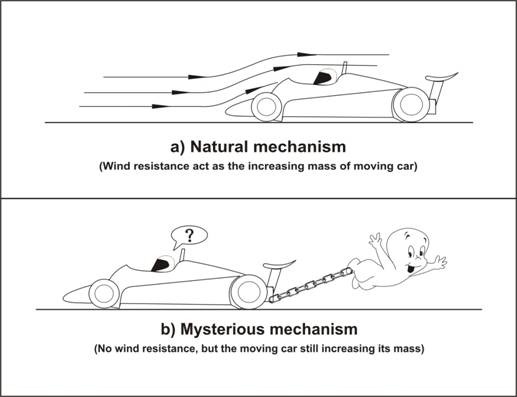Fig.1. Magic vs. realistic mechanism of increasing of mass.

Armed with a new concept of vacuum mechanics (mechanism of vacuum medium) we could improve the theory, which make it possible to explain the mechanism of the increasing mass. Indeed we will found that it is not a real increasing mass, instead it is just the effect arisen from reactive resistance of vacuum medium!

1) This paper is talking about “the mechanism of increasing mass” of any moving object in which there is no explanation from the conventional interpretation in STR. It is an extension of the paper “Completed Einstein special theory of relativity (CSTR)” present in this website (www.vacuum-mechanics.com). In CSTR we have show how to prove by using a simple scientific experiment (two-solenoid experiment) for the existence of “vacuum medium” instead of the conventional empty space according to STR.

2) First let us start with the diagram show in fig.1 (a), which will give us an easy way to visualize how to interpret the concept of the increasing mass of the moving object. In our daily life for car driving experience, in which we will found that at low speed the fuel consumption rate, is less than at high speed, the faster car - the higher consumption rate. The reason is because of air’s resistance, the faster speed - the higher resistance.

Now we could interpret the dragging force of the surrounding air (which acts on a moving car, a flying plane etc.) as the mechanism for the increasing mass of the moving object.   Indeed we could see that the faster moving car means the greater increasing mass of the car!

Note suppose that we are driving in an air free tunnel without air’s resistance, then we would found that the fuel consumption rate is constant at any speed. This means that the moving car’s mass is constant too! This is the concept of Galilean transformation which works under the concept of Newton absolute empty space!

3) Next let us consider diagram show in fig.1 (b) which represents the conventional concept of the increasing mass according to STR. Here the concept based on of empty space, so we do not know why and how it gives the increasing mass like that!

To see the mechanism of the increasing mass we have to improve STR, based on the concept of vacuum medium as explained in CSTR. In which we have derived a mathematical formula for the increasing mass by using the analogy of Lienard-Wiechert potential of a “moving” charge (in electrodynamics) and summarized as below;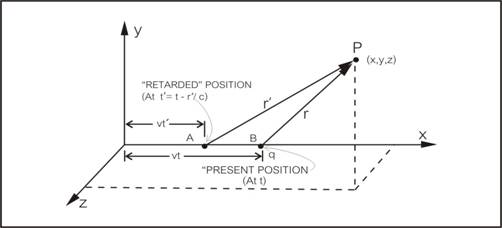Fig. 2. A moving charge’s potential with constant velocity.

Consider the Lienard-Wiechert potential of a “moving” charge as in the equation (1) shown in the diagram fig. 2,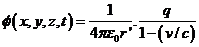……. (1),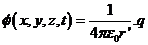…… (2).

When we compare it to a “static” electric potential of a stationary charge (2) above, we would found that the moving charge appeared to “increase its charge” by a factor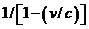which is equivalent to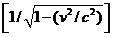in its present position in (2).

And we known that gravity has the same attraction rule as Coulomb law in electricity, if we replace the charge q with a mass then we would found that its gravitation potential is the same form as electric potential above. So the “static” gravitational potential of a stationary mass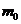is as (3), while the “moving” gravitational potential of the moving mass is as (4) below.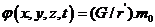…. (3),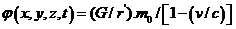….. (4).

Then we could found the gravitational potential at P in the present coordinate, in the same way as the moving charge, and we get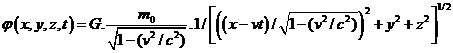….. (5).

Lastly, we could see that the moving mass appeared to increase its mass by a factoras in (4) or equivalent toas in (5)  ( in the same way as a “moving” charge do as showed above)!

4) Now we will consider what the “increasing mass” is. From the gravitational potential in equation (3) and (5) we could change it to the “action force F acts at a unit of test mass at P by squaring the distance in the dominator as in equation (6) and (7) below.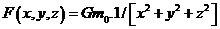…………………………………….. (6).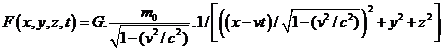….. (7).

Next, in classical mechanics where Newton gravitational law and Galilean transformation were used for equation (28) then it will change to equation (29) below.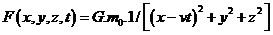…………. (8).

When comparing equation (7) to equation (8) we would found that the moving mass seems to “increase its intrinsic mass” without any cause!

Finally, if we accept that the mass is immerged in vacuum medium, then we could understand that the “increasing of mass” represent the “additional mass” due to the “resistive action of vacuum medium” to the moving mass!

5) Next let us see an example which shows how the conventional concept (of STR) was used to explain how the increasing mass works. The diagram show in fig. 3 is talking about relativistic momentum that involved the collision between two elastic particles. [Reference: D. Halliday, R. Resnick, and K. Krane, text book “Physics” Vol. 1 Extended Version Fourth Edition, p.482-483.]

In (a) the situation before the collision in the reference frame S, (b) after the collision in S, (c) the situation before the collision in the reference frame S’ and (d) after the collision in S’.

Using the classical (non-relativistic) formula, the components of the momentum of the two-particle system in S are

Initial;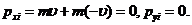Final;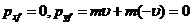.

Thus the momentum is conserved!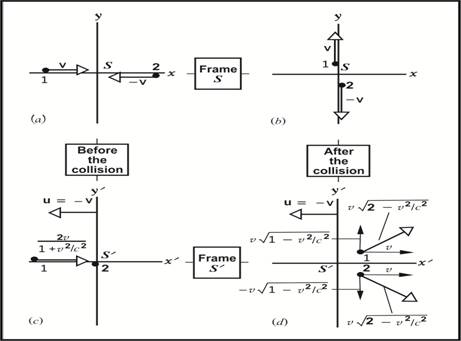Fig. 3. Collision between two elastic particles with same mass.

Next, when viewing the same collision from the S’ frame which move relative to S with speed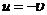. Together with using Lorentz velocity transformation for x’ and y’ components observed by S’ that are shown in (c) and (d), the momentum of the system are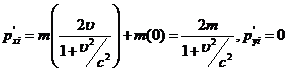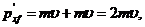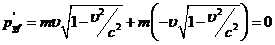. ….(9)

Then here, clearly the momentum is not conserved.

But if we use the relativistic formulas of momentum,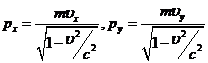then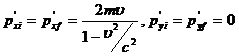…….(10)

So this means the momentum is conserved. And to solve this problem, relativistic momentum was invented. But notice that there is no explanation why the momentum (9) in S’ is not conserved.

Finally when both masses collide with each other then we will get the result as in (10). To understand equation (10) we have to rewrite it as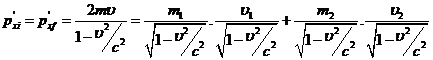…… (11)

(Where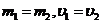.) Looking at the right hand side, the first and third terms are what be called relativistic mass, while the second and forth terms are the speeds of force field from both of the moving masses. Anyway, STR cannot explain why and how both the moving masses get their additional mass.

In contrary, armed with our new concept of vacuum mechanics (mechanism of vacuum medium), we could understand that the “additional mass” is the effect of the “resistive reaction of vacuum medium” because the moving masses were immerged in vacuum medium as mentioned early.

6) Finally let us talk about the word “relativistic” in STR, such as relativistic mass and relativistic momentum which we just have seen. Conventionally it means that some physical quantity of the moving object which changed from its “rest” value.

Note that both relativistic mass and relativistic momentum are increasing their value while the mass is moving. And we could see that their increased value corresponds to the resistive reaction of vacuum medium as mentioned early. Indeed it is not the real increasing of its intrinsic mass or momentum!

Next, we have two more relativistic effect i.e. length contraction and time dilation. For length contraction, one who familiar with STR would found that it has no obvious prove about it. Then the question is it a real physical length contraction? And could vacuum mechanics concept explain how and why it is?

Finally, for time dilation, although there have been obviously proved, such as by comparing the time measured in a flying jet plane around the world with the time measured on the earth. Again there is also the question of time dilation that is it a real slowing of time itself or anything else? In the next paper “What really the time dilation in relativity means?” we will show how our CSTR could answer both the two mentioned problems.

…………………………………….